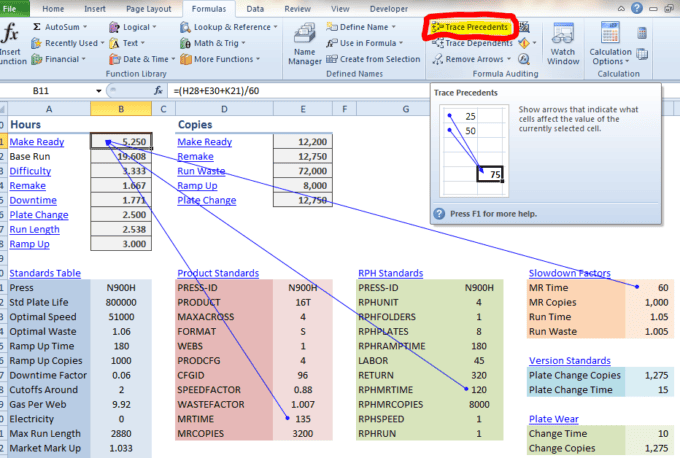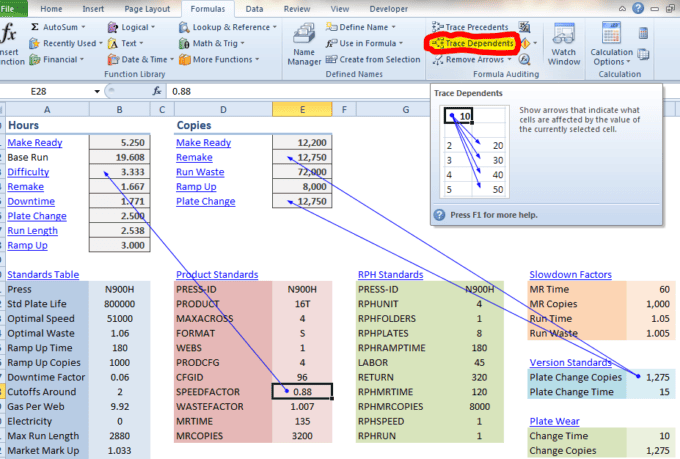Use Formula Auditing to Help Explain Formulas Excel

When you need to present a complicated spreadsheet, the Formula Auditing feature in Excel visually helps to explain formula relationships. You can easily trace formula precedents or dependents.

Think of precedents as “what comes before” or the inputs to a formula. If you need to show a formula precedent select a cell with a formula, then choose Formulas > Trace Precedents to show where the formula inputs are coming from.Think of formula dependents as the values that feed a formula. Below you see two different cells tracing dependents. Both cells have a constant values that support, or directly influence three different formulas.

To trace a dependent, select a cell then choose Formulas > Trace Dependents.By using the Formula Auditing feature in Excel, you’re sure to give a visual look and hopefully a better understanding of the relationship between formulas and values in a spreadsheet.1 thought on “Use Formula Auditing to Help Explain Formulas Excel”

1.outsource excel

This is by far and away my favorite feature of Excel 2007(+). Now, when you have a complicated formula (that is based off the values of dozens of other cells), it’s easy to adjust the input values and see what sort of effect they have on the outputs.